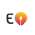### MW transmitter using Arduino nano

MW transmitter using Arduino nano

Circuit Diagram
MW frequency :- Medium wave is the part of the medium frequency radio band used mainly for AM radio broadcasting. The spectrum provides about 120 channels with limited sound quality. During daytime, only local stations can be received.

Code

_____________________________________________

#define ANTENNA_PIN PB3
void setup() {
Serial.begin(115200);
uint32_t fTransmit = 600;
DDRB |= (1 << ANTENNA_PIN);
TCCR2A = (0 << COM2A1) + (1 << COM2A0);
TCCR2A |= (1 << WGM21) + (0 << WGM20);
TCCR2B = (0 << CS22) + (0 << CS21) + (1 << CS20);
OCR2A = F_CPU / (2000 * fTransmit) - 1;

char strbuf;

sprintf(strbuf, "Will broadcast at %d KHz", (F_CPU / (2 * (1 + OCR2A)) / 1000));
Serial.println(strbuf);
TCCR1A |= (1 << WGM11) + (1 << WGM10);
TCCR1B = (1 << WGM12);
TCCR1B |= (0 << CS12) + (0 << CS11) + (1 << CS10);
TIMSK1 = (1 << OCIE1A) + (1 << TOIE1);
ADMUX = (1 << REFS1) + (1 << REFS0);
}

ISR(TIMER1_OVF_vect) {

DDRB |= (1 << ANTENNA_PIN);
}

ISR(TIMER1_COMPA_vect) {

DDRB &= ~(1 << ANTENNA_PIN);
}

void loop() {

}
_____________________________________________

1.1.